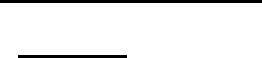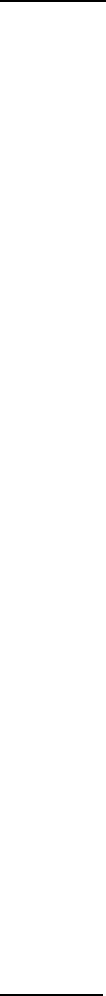# MA121 Study Guide - Midterm Guide: Logical Consequence, Boolean Data Type, Commutative Property

168 views38 pages
School
Department
Course
Professor

Wilfrid Laurier University
MA121
Introduction to Mathematical Proofs
Fall 2017
Term Test 1
Prof: Robert John Rundle
Exam Guide
Unlock document

This preview shows pages 1-3 of the document.
Unlock all 38 pages and 3 million more documents.TOPICS COVERED
CHAPTER 1: SETS
1.2 Elements and Sets
1.3 Venn Diagrams
1.4 Unions
1.5 Intersections
1.5 Complements
1.6 Set Difference
CHAPTER 2: PROPOSITIONAL LOGIC
2.2 Statements, Connectives, Truth Tables
2.3 Equivalences of Statements
2.4 Construction of Compound Statements
2.5 Valid Arguments
2.6 Methods of Proof: Direct Proof of Implications, Methods of Proof of Implications:
Contrapositive, Contradiction; Proof by Cases, Disprove by Counterexamples
2. 7 Propositions and Predicates
2. 8 Quantified Statements, Constructive and Non-constructive Proofs
2.9 Proof by Induction
CHAPTER 1: SETS
1.2 ELEMENTS AND SETS
1.2.1 Definition A set is a collection of objects together with some rule to determine whether a
given object belongs to this collection. Any object of this collection is called an element of the
set.
Notation: The name of a set is denoted with a capital letter A, B, etc.
The description of the set can be given in the following ways:
1. Each element of the set is listed within a set of brackets: { }.
2. Within the brackets, the first few elements are listed, with dots following to show that the set
continues with the selection of the elements following the same rule as the first few.
3. Within the brackets, the set is described by writing out the exact rule by which elements are
chosen. The name given each element is separated from the selection rule with a vertical
line.
Unlock document

This preview shows pages 1-3 of the document.
Unlock all 38 pages and 3 million more documents.1.2.2 Examples:
a) The set of all even numbers
b) The set of all books written about travel to Chile
c) Denote by A the set of natural numbers with are greater than 25. The set could be written
in the following ways: {26, 27, 28….} (using the second notation listed above)
{x | x is a natural number and x > 25} (using the third notation above)
The above description is read as “the set of all x such that x is a natural number and
x > 25”.
Note that 32 is an element of A. We write 32 A, where “” denotes “is an element of.”
Also, 6 A, where “” denotes “is not an element of.”
d) Let B be the set of numbers {3, 5, 15, 19, 31, and 32}. Again the elements of the set are
natural numbers. However, the rule is given by actually listing each element of the set
(as in the first notation above). We see that 15 B, but 23 B.
e) Let C be the set of all natural numbers which are less than 1. In this set, we observe that
there are no elements. Hence, C is said to be an empty set.
A set that contains no elements, { }, is called the empty set and is notated
A universal set is a set that contains all the elements we are interested in. This would have to be
defined by the context
A set simply specifies the contents; order is not important. The set represented by {1, 2, 3} is
equivalent to the set {3, 1, 2}.
1.2.3 Definition: A set A is said to be a subset of a set B if every element of A is an element of
B.
Notation: To indicate that set A is a subset of set B, we use the expression A B, where “
denotes “is a subset of”. A B means that A is not a subset of B.
Unlock document

This preview shows pages 1-3 of the document.
Unlock all 38 pages and 3 million more documents.